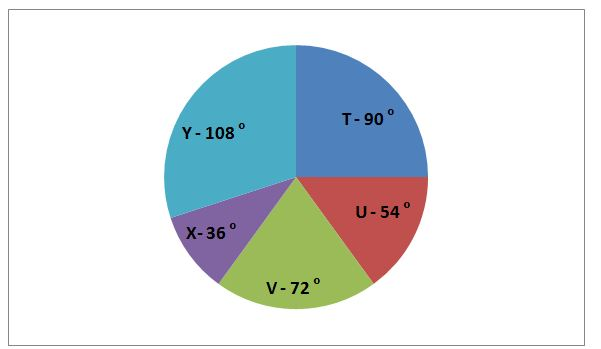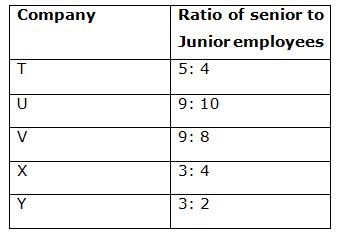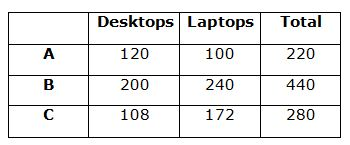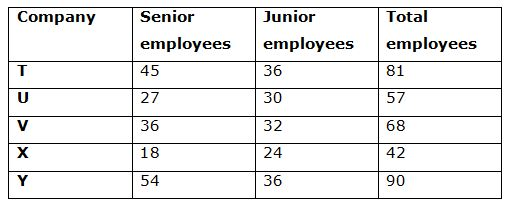# LIC HFL Assistant Manager Quantitative Aptitude Questions – (Day-04)

Dear Aspirants, Our IBPS Guide team is providing new series of Quantitative Aptitude Questions for LIC HFL Assistant Manager 2019 so the aspirants can practice it on a daily basis. These questions are framed by our skilled experts after understanding your needs thoroughly. Aspirants can practice these new series questions daily to familiarize with the exact exam pattern and make your preparation effective.

Check here for LIC HFL Mock Test 2019

Check here for IBPS RRB PO Mains Mock Test 2019

Check here for IBPS RRB Clerk Mains Mock Test 2019

### Click Here to Subscribe Crack High Level Puzzles & Seating Arrangement Questions PDF 2019 Plan

[WpProQuiz 7021]

Directions (1 – 5): Read the following information carefully and answer the given question.

There are three companies A, B and C sold laptops and desktops in January 2019.

Number of laptops sold in company C is 28 less than the number of desktops sold in company B. Number of laptops sold in company B is 240, which is 20% more than the number of desktops sold in the same company.

Total number of items sold in company A is 50% of the total number of items sold in company B. Number of desktops sold in company A is half of the number of laptops sold in company B.

The ratio of the number of laptops sold in company A to that of the number of desktops sold in company C is 25: 27.

1) Number of laptops sold in company A is what percentage more/less than number of desktops sold in company B?

a) 50% more

b) 30% less

c) 40% more

d) 50% less

e) None of these

2) What is the ratio of the total number of desktops sold in all the companies together to that of the total number of items sold in company C?

a) 113: 79

b) 119: 73

c) 117: 70

d) 103: 79

e) None of these

3) What is the difference between the total number of items sold in company A to that of C?

a) 40

b) 60

c) 30

d) 50

e) 72

4) If company D sold number of desktops is 20% more than that of company A and the ratio of the number of desktops sold in company D to that of laptops is 2: 3. What is the total number of items sold in company D?

a) 360

b) 240

c) 300

d) 320

e) 400

5) If 20% of the laptops sold in company B is defective, find the number of non – defective laptops sold in company B?

a) 216

b) 180

c) 196

d) 172

e) 192

Directions (6 – 10): Study the following graph carefully and answer the given questions.

The pie chart shows the distribution of total number of senior employees in different companies.

Number of senior employees in company X = 186) What is the percentage of number of senior employees in company U?

a) 20%

b) 18%

c) 21%

d) 15%

e) 28%

7) Find the total number of junior employees in company T, U and X together?

a) 70

b) 75

c) 60

d) 50

e) 90

8) If total number of female employees in company Y is 40 and the number of junior male employees in company Y is 20, then find the total female senior employees in the same company?

a) 12

b) 36

c) 24

d) 48

e) 20

9) If the number of senior employees in company S is 25% more than company V and the ratio of senior to junior employees in company S is 3: 4. Number of junior employees in company S is what percent more/less than the number of junior employees in company U?

a) 20%

b) 40%

c) 60%

d) 50%

e) 100%

10) Which of the following company has maximum number of employees (Senior + Junior)?

a) X

b) U

c) T

d) Y

e) V

Directions (1 – 5):

Number of laptops sold in company B = 240

Number of desktops sold in company B = 240 * (100/120) = 200

Number of laptops sold in company C = 200 – 28 = 172

Total number of items sold in company B = 240 + 200 = 440

Total number of items sold in company A = 440 * 50/100 = 220

Number of desktops sold in company A = ½ * 240 = 120

Number of laptops sold in company A = 220 – 120 = 100

Number of desktops sold in company C = 100 * (27/25) = 108Required percentage = (200 – 100)/200 * 100

= 100/200 * 100 = 50% less

Required ratio = (120 + 240 + 108): 280

= 468: 280

= 117: 70

Required ratio = 280 – 220 = 60

Number of desktops sold in company D = 120 * (120/100) = 144

Number of laptops sold in company D = 144 * (3/2) = 216

Required total = 144 + 216 = 360

Required number of laptops = 240 * 80/100 = 192

Directions (6 – 10):Required percentage = 54˚/360˚ * 100

= 15%

Required total = (36 + 30 + 24) = 90

Total number of employees = 54 + 36 = 90

Total number of female employees = 40

Total number of male employees = 90 – 40 = 50

Number of senior male employees = 50 – 20 = 30

Number of senior female employees = 54 – 30 = 24

Number of senior employees in company S = 125/100 * 36 = 45

Number of junior employees in company S = 45/3 * 4 = 60

Required percentage = (60 – 30)/30 * 100 = 30/30 * 100 = 100%

From company T = 45 + 36 = 81

From company U = 27 + 30 = 57

From company V = 36 + 32 = 68

From company X = 18 + 24 = 42

From company Y = 54 + 36 = 90

Company Y has maximum number of employees.